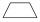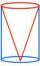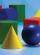# Geometry - math word problems

#### Number of problems found: 1476

• Isosceles trapeziumTrapezoid YUEB (YU||EB) is isosceles. The size of the angle at vertex U is 49 degrees. Calculate the size of the angle at vertex B.
• PresentGift box has a rectangular shape with dimensions of 8×8×3 cm. Miloslav wants to cover with square paper with sides of 18 cm. How much paper left him?
• Cone in cylinderThe cylinder is inscribed cone. Determine the ratio of the volume of cone and cylinder. The ratio express as a decimal number and as percentage.
• Cone in cubeThe cube is inscribed cone. Determine the ratio of the volume of cone and cube. The ratio express as a decimal number and as percentage.
• AquariumAquarium is rectangular box with square base containing 76 liters of water. Length of base edge is 42 cm. To what height the water level goes?
• Cube wallThe surface of the first cube wall is 64 m2. The second cube area is 40% of the surface of the first cube. Determine the length of the edge of the second cube (x).
• Cube in ballCube is inscribed into the sphere of radius 181 dm. How many percent is the volume of cube of the volume of the sphere?
• Hollow sphereCalculate the weight of a hollow stříbrné sphere (density 10.5 g/cm3) if the vnitřní diameter is 15 cm and wall thickness is 1 mm.
• Cube - anglesCalculate angle between the wall diagonal and cube base. Calculate the angle between the cube body diagonal and cube base.
• Plasticine ballPlasticine balls have radius r1=85 cm, r2=60 mm, r3=59 cm, r4=86 cm, r5=20 cm, r6=76 mm, r7=81 mm, r8=25 mm, r9=19 mm, r10=14 cm. For these balls
• Cube diagonalDetermine the length of the cube diagonal with edge 37 mm.
• SandpileAuto sprinkled with sand to an approximately conical shape. Workers wanted to determine the volume (amount of sand) and therefore measure the base's circumference and the length of both sides of the cone (over the top). What is the sand cone's volume if t
• Rotation of the EarthCalculate the circumferential speed of the Earth's surface at a latitude of 61°​​. Consider a globe with a radius of 6378 km.A passenger car and an ambulance come to the rectangular crossroad, the ambulance left. Passenger car at speed 39 km/h and ambulance at 41 km/h. Calculate such a relative speed of the ambulance move to the car.Eiffel Tower in Paris is 300 meters high and is made of steel. Its weight is 8000 tons. How tall is the tower model made ​​of the same material if it weighs is 2.4 kg?Rudolf decided to take the train from the station 'Ostratice' to 'Horné Ozorovce'. In the train timetables found train Os 5409 : km 0 Chynorany 15:17 5 Ostratice 15:23 15:23 8 Rybany 15:27 15:27 10 Dolné Naštice 15:31 15:31 14 Bánovce nad Bebravou 15:35 1